Faraday Constant and Avogadro Number Lab Report

# INTRODUCTION

Zn/Cu Voltaic Cell: In a voltaic cell consisting of Zn and Cu electrodes as shown in Fig. 1, Zn is the stronger reducing agent than Cu and hence will be oxidized as it reduces Cu2+ ion to metallic Cu. The Zn electrode is the negatively-labelled anode and the Cu electrode is the positively-labelled cathode. Electron current flows spontaneously through an external circuit from the Zn electrode to the Cu electrode.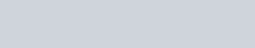Figure 1

Zn/Cu Electrolytic Cell: When a battery is wired to the external circuit that connects the Zn and Cu electrodes, battery power can be applied to cause a current to flow in reverse from the Cu electrode to the Zn electrode as shown in Fig. 2. This is now an electrolytic cell.

1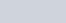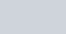e

e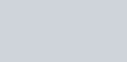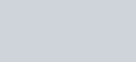Cu(s)

Cu

2+

+

2 e

Zn

2+

+

2 e

Zn(s)

Figure

2

In an electrolytic cell, the current flow is nonspontaneous and requires a continuous input of an external source of power to occur. Unlike a voltaic cell, the anode of an electrolytic cell is labelled as positive electrode and the cathode as negative electrode. Electrolysis has many applications, including plating of metals on surfaces and production of pure elements from mining ores or other compounds.

As in a voltaic cell, oxidation occurs at the anode and reduction occurs at the cathode in an electrolytic cell. The amount of reaction that occurs at the electrodes is directly proportional to the number of electrons transferred. Michael Faraday (1791-1867) determined that the mass of a substance produced or consumed at the electrodes during electrolysis is proportional to the quantity of charge [Charge (C) = Current (A) x Time (sec)] that has passed through the circuit. A faraday is defined as the total charge (in Coulombs) on 1 mole of electrons and is equal to 96,485 C. Hence, the charge on a single electron is 1.602 x 10−19 C.

In this electrolysis experiment, you will determine (1) the experimental value of the Faraday’s Constant by measuring the amount of charge required to consume a known mass of copper on an electrode. From the mass of copper consumed (or electrolyzed), you will also be able to determine (2) the experimental value of the Avogadro’s number (NA).

You will use a copper strip and a zinc strip as the electrodes. These are placed in a beaker of dilute sulfuric acid (H2SO4) as shown in Fig 3. The strong acid dissociates into ions in solution and thus allows a current to be conducted between the electrodes. A Direct-Current (DC) Power Supply is used as a source of power to drive the electrolytic cell. Using the DC power supply, an electric current of specific amperage can be set.

At the anode, the following oxidation reaction takes place:DC power

supply

e

a

nod

e

cathod

e

Figure

3

Cu(s) → Cu2+(aq) + 2 e

By determining the average current used in the reaction, along with the knowledge that all copper ions formed are the 2+ cations, you will calculate the number of atoms in one mole of copper (i.e. experimental Avogadro’s number, NA) and compare this value with the accepted Avogadro’s number (NA

= 6.022 x 1023).

# PROCEDURE

A description of the procedure and sample calculations are given below to help you understand how the data are generated and used to achieve the objectives of this lab.

1. Two dry metal strips, one is made of copper and the other of zinc, are cleaned by scrubbing their surface with steel wool to remove any surface oxidation. The Cu dry metal strip is placed in an analytical balance and its mass recorded.

1. The metal strips are connected to a DC Power Supply via wires with alligator clips as shown in Fig. 4. The Cu metal strip acts as the anode and is connected to the Power

Supply at the positive (+) terminal. The Zn metal strip acts as the cathode and is connected to the Power Supply at the negative (‒) terminal. [Note: In an electrolytic cell, the anode is where electrons are pulled out of the electrode into the Power Supply and is given a (+) sign, and the cathode is where electrons are pushed out of the Power Supply and flow toward].

The sample initial dry masses of the Zn and Cu strips are provided on the diagram, (which I borrowed from an internet source for illustration). The mass of the Zn strip and the voltage shown are irrelevant for this lab. The relevant data are the initial mass of the Cu strip (17.3527 g), the current used (0.4004 A), and the total time (3.00 minutes) of electrolysis.Figure

4

17.3527

g

17.

8551

g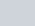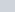+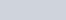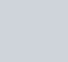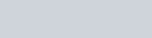1

M H

2

SO

4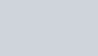The Electrolytic Cell

1. The metal electrodes are placed in 1 M H2SO4 contained in a large glass beaker and are set far apart from one another to prevent accidental contact. The experiment is performed in 3 trials, each time the electrodes are immersed in the H2SO4 solution to equal depths. The Power Supply is adjusted to deliver a current within the range of 0.2‒0.6 A to the cell for a period of exactly 3.00 minutes. In the sample trial shown in Fig 4, the current recorded as 0.4004 A.

1. After 3 minutes of electrolysis, the power is turned off. The Cu strip is removed and rinsed in distilled H2O using a wash bottle, and gently blotted dry with paper towels. After being allowed to air dry completely, the Cu strip is placed in the analytical balance and its mass recorded.

# DATA ANALYSIS AND SAMPLE CALCULATIONS

During electrolysis, the Cu electrode is the anode and therefore oxidized: Cu(s) → Cu2+ + 2e As a result of oxidation, its mass decreases from the initial 17.3527 g to 17.3247 g.

Mass of Cu lost (or electrolyzed) = 17.3527 g ‒ 17.3247 g = 0.0280 g

Average current used = 0.4004 A

Total time of electrolysis = 3.00 minutes x 60 s / min = 1.80 x 102 s (3 sig figs)

Total charge transferred: Charge (C) = Current (A) x Time (sec)

= 0.4004 A x 1.80 x 102 s

# = 72.1 C

Number of e produced based

on the total charge transferred : 72.1 C x 1 e = 4.50 x 1020 e

1.602 x 10‒19 C

Mole of e produced based on

the mass of Cu electrolyzed : 0.0280 g x 1 mole Cu x 2 mole e = 8.81 x 10‒4 mol e

63.55 g Cu 1 mole Cu

# Experimental

Faraday’s Constant : Total charge (C) = 72.1 C = 81,839 C/mol e mole e 8.81 x 10‒4 mol e

# 81.8 x 103 C/mol e‒

(3 sig figs)

Number of Cu atoms lost

from anode electrode : 4.50 x 1020 e x 1 Cu atom = 2.25 x 1020 Cu atoms

2 e

Number of Cu atoms lost

per gram Cu electrolyzed : 2.25 x 1020 Cu atoms = 8.04 x 1021 Cu atoms

0.0280 g Cu g Cu

Number of Cu atoms per

mole of Cu : 8.04 x 1021 Cu atoms x 63.55 g Cu = 5.11 x 1023 Cu atoms

 same as g Cu mol Cu mol Cu

Your tasks: The experiment as described is performed in 3 trials, and the relevant data are tabulated in the data table presented on page 5. Perform the necessary calculations as shown above to complete the table. Since you’re not performing the actual lab, your calculation results will be heavily weighed. Particular attention should be given to correct sig figs and proper units when data are entered into the table for full credit. 0.2 pt will be deducted for each of these mistakes.

In the blank space provided on page 6, show sample calculation set ups for data of trial 1. Include calculation set ups for percent error and standard deviation. Attach another piece of paper is extra space is needed.

DATA TABLE: FARADAY’S CONSTANT & AVOGADRO’S NUMBER

 Trial 1 Trial 2 Trial 3 Initial mass of Cu electrode 17.32 41 g 17.2928 g 17.2709 g Final mass of Cu electrode 17.2993 g 17.2675 g 17.2475 g Mass of Cu lost Average current 0.4065 A 0.4028 A 0.3911 A Time of current application 1.80 x 102 s 1.80 x 102 s 1.80 x 102 s Total charge transferred Number of e− produced based on total charge transferred Mole of e− produced based on mass of Cu electrolyzed Experimental Faraday’s Constant Ave. experimental Faraday’s Constant Standard deviation of Faraday’s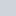Constant (same sig figs as ave. #) Percent error (use ave. value in calculation)(rounded off to whole integer; if < 1, write 0%)[Accepted value for Faraday’s = 96485 C/mol e−] Number of Cu atoms lost from anode electrode Number of Cu atoms lost per g Cu electrolyzed Number of Cu atoms per mole Cu[Experimental Avogadro’s Number = (NA)] Ave. Experimt Avogadro’s Number (NA) Standard deviation of Experimental NA(same # sig figs as ave. #) Percent error (use ave. value in calculation)(accepted value: 6.022 x 1023)

SAMPLE CALCULATIONS FOR DATA OF TRIAL 1. INCLUDE CALCULATION SET UPS FOR PERCENT ERROR AND STANDARD DEVIATION.

# POST-LAB QUESTION [ Note: Show all calculation set ups leading to the correct answers to receive credit.]

1. (2 pts) Electrolysis of a solution of CuSO4(aq) to give Cu metal is carried out using a current of 0.66 A. How long, in minutes, should electrolysis continue to produce 0.50 g of copper?

1. (2 pts) In the electrolysis of a solution containing Ni2+(aq), metallic Ni(s) deposits on the cathode. Using a current of 0.150 A for 12.2 minutes, what mass, in g, of nickel will form ?